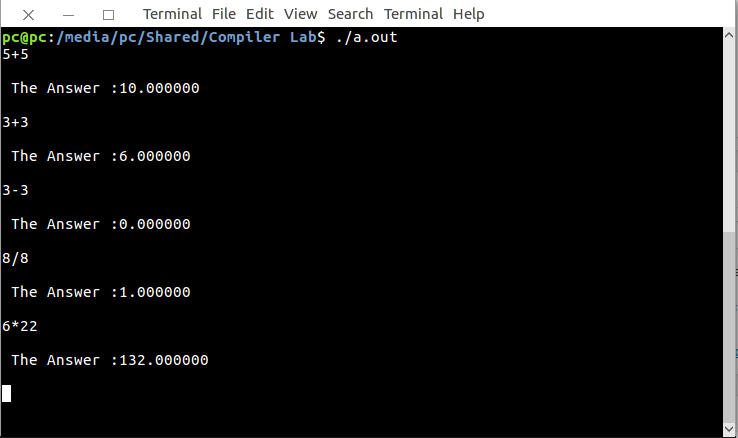# Lex program to implement a simple Calculator

Lex is a computer program that generates lexical analyzers. Lex reads an input stream specifying the lexical analyzer and outputs source code implementing the lexer in the C programming language.

The commands for executing the LEX program are:

```lex abc.l (abc is the file name)
cc lex.yy.c -efl
./a.out ```

Let’s see LEX program to implement a simple calculator.

Examples:

```Input :
3+3
Output :
6.0

Input :
5*4
Output :
20.0 ```

Below is the implementation:

 `/*lex program to implement  ` `         ``- a simple calculator.*/` ` `  `% { ` `  ``int` `op = 0,i; ` `  ``float` `a, b; ` `% } ` ` `  `dig [0-9]+|([0-9]*)``"."``([0-9]+) ` `add ``"+"` `sub ``"-"` `mul ``"*"` `div` `"/"` `pow` `"^"` `ln \n ` `%% ` ` `  `/* digi() is a user defined function */` `{dig} {digi();}  ` `{add} {op=1;} ` `{sub} {op=2;} ` `{mul} {op=3;} ` `{``div``} {op=4;} ` `{``pow``} {op=5;} ` `{ln} {``printf``(``"\n The Answer :%f\n\n"``,a);} ` ` `  `%% ` `digi() ` `{ ` ` ``if``(op==0) ` ` `  `/* atof() is used to convert  ` `      ``- the ASCII input to float */` ` ``a=``atof``(yytext);  ` ` `  ` ``else` ` ``{ ` ` ``b=``atof``(yytext); ` ` `  ` ``switch``(op) ` ` ``{ ` `   ``case` `1:a=a+b; ` `    ``break``; ` ` `  `   ``case` `2:a=a-b; ` `   ``break``; ` `  `  `   ``case` `3:a=a*b; ` `   ``break``; ` `  `  `   ``case` `4:a=a/b; ` `   ``break``; ` `  `  `   ``case` `5:``for``(i=a;b>1;b--) ` `   ``a=a*i; ` `   ``break``; ` `  ``} ` ` ``op=0; ` ` ``} ` `} ` ` `  `main(``int` `argv,``char` `*argc[]) ` `{ ` ` ``yylex(); ` `} ` ` `  `yywrap() ` ` ``{ ` `  ``return` `1; ` ` ``} `

Output:Attention reader! Don’t stop learning now. Get hold of all the important DSA concepts with the DSA Self Paced Course at a student-friendly price and become industry ready.

My Personal Notes arrow_drop_upCheck out this Author's contributed articles.

If you like GeeksforGeeks and would like to contribute, you can also write an article using contribute.geeksforgeeks.org or mail your article to contribute@geeksforgeeks.org. See your article appearing on the GeeksforGeeks main page and help other Geeks.

Please Improve this article if you find anything incorrect by clicking on the "Improve Article" button below.